### SOLUTIONS TO DIFFERENTIATION OF FUNCTIONS USING THE PRODUCT RULE

SOLUTION 1 : Differentiate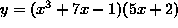.

Then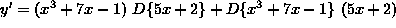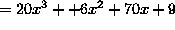.

SOLUTION 2 : Differentiate.

Then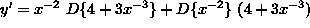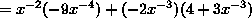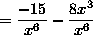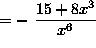.

SOLUTION 3 : Differentiate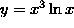.

Then.

SOLUTION 4 : Differentiate.

Then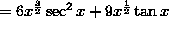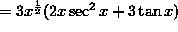.

SOLUTION 5 : Differentiate.

Then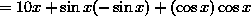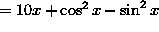(An alternate answer can be given using the trigonometry identity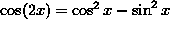.)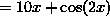.

SOLUTION 6 : Differentiate.

Then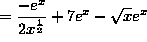.

SOLUTION 7 : Differentiate.

Then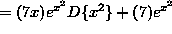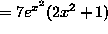.

SOLUTION 8 : Differentiate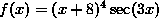.

Then.

SOLUTION 9 : Differentiate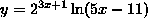.

Then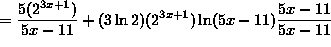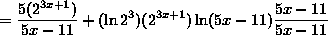.

SOLUTION 10 : Differentiate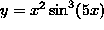.

Then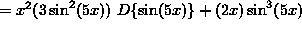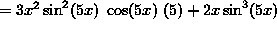.

SOLUTION 11 : Differentiate.

Then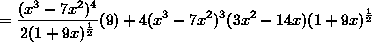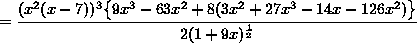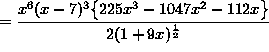.

SOLUTION 12 : Differentiate.

Then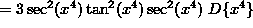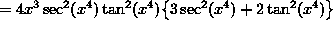.